# Resolved - Issue with dual 74hc595 output from Arduino

#### Remembermyname

Joined Sep 6, 2015
91
Greetings,
I recently completed a project involving reading temperatures with an Arduino that uses 4x seven segment LED displays. Currently, I am attempting to develop a similar system which uses a single segment decimal display likened to the function of a nixie (without the high voltage) or an edge-lit numerik display where the display digit consists of a column of 10 LED's (0-9), a decimal point LED and an LED that indicate a negative sign for a given digit in the display.

I attempted to build this from a previous project which can be found at the following:

For now, the circuit is only built to simply display a number set in a variable in the code only in order to minimize troubleshooting but I've run into an issue that I'm having trouble resolving. The circuit was changed to suit two 74HC595's to drive 12 LED's per column. Each column is then multiplexed. The circuit schematic is pasted below: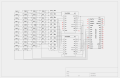This is the circuit. It is run by an Arduino Nano driving two 74HC595's cascaded. The lower bit 595 is on the right closest to the Arduino. The higher bit to the left in the image below: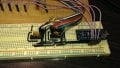To view the output in decimal format, a temporary display head was built on a perfboard. There are four columns, one for each digit with the MSD on the left, LSD to the right: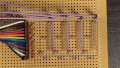On each digit, a column of 10 LED's are used counting 0-9 with a decimal point at the bottom and a negative indicator to the left of each digit column: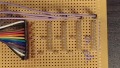Once in operation, the display indicates the number almost correctly (haven't worked on the decimal point portion of the code yet) - in this case the number 123.4 . The first 595 is working correctly. It is handling the first 8 of 16 bits for the digit LED's 0-7. The second 595 on the other hand is not. It is handling the second 8 of 16 bits for the digit LED's 8-9 , decimal point and negative indicator. The second 595 dimly lights it's LED's. It does however brightly activate it corresponding LED's if I give the number an 8 or a 9. I am having the issue of the second 595 deactivate the LED's when not in use. I have been looking around the interwebs trying to find what is going on with it. I've changed the 595 with different one and rewired with new wire. I've added .1uF capacitors to decouple the power and ground pins of the 595's with no change. I've poked and prodded about the circuit thinking that it might be radio noise but no changes of any kind occur. So I looked at the code. I'm having trouble knowing what to appropriately search for as I have an inclination that the problem lies with the code in the 'void refreshDisplay()' routine. I haven't worked with cascaded 595 before. Poking around the code in this area have given (albeit unpredictable and strange) changes. The current operation of the display is in the image below: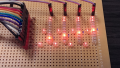There are many articles on how to use the 595's in this fashion but I must have missed or misunderstood something. I'm fairly certain that I'm doing something wrong. Does anyone know of a proper solution to this or may know the correct way to operate this? Any help would be greatly appreciated. The current code for this circuit is pasted below:

C:
/*Original code by:
* http://www.pial.net/arduino-controlling-a-4-digit-seven-segment-display/ ( but modified somewhat)
*/
//#include <OneWire.h>
//#include <DallasTemperature.h>
//#define ONE_WIRE_BUS 2
//OneWire oneWire(ONE_WIRE_BUS);
//DallasTemperature sensors(&oneWire);
const int ledPin =  13;// LED connected to digital pin 13
const int latchPin = 8;//Pin connected to ST_CP of 74HC595
const int clockPin = 9;//Pin connected to SH_CP of 74HC595
const int dataPin = 10;//Pin connected to DS of 74HC595
const int digitPins = {
3,4,5,6}; //pins to control the 4 common anode pins of the display

const byte COL_COUNT = 12;
unsigned int digit[COL_COUNT] = //single decimal digit bits + blank + minus
{
0B0000000000000001,  // 0
0B0000000000000010,  // 1
0B0000000000000100,  // 2
0B0000000000001000,  // 3
0B0000000000010000,  // 4
0B0000000000100000,  // 5
0B0000000001000000,  // 6
0B0000000010000000,  // 7
0B0000000100000000,  // 8
0B0000001000000000,  // 9
0B0000000000000000,  // all segments off
0B0000010000000000,  // -
//  0B0000100000000000,  // .
};
int digitBuffer = {
1};
int digitScan = 0;
int soft_scaler = 0;
float tempC, tempF;
int tmp;
boolean sign = false;
void setup()  {
TCCR2A = 0;
TCCR2B = (1<<CS21);
TIMSK2 = (1<<TOIE2);
TCNT2 = 0;
Serial.begin(9600);
pinMode(ledPin, OUTPUT);
for(int i=0;i<4;i++)
{
pinMode(digitPins[i],OUTPUT);
}
pinMode(latchPin, OUTPUT);
pinMode(clockPin, OUTPUT);
pinMode(dataPin, OUTPUT);
//  sensors.begin();
}
ISR(TIMER2_OVF_vect) {
soft_scaler++;
if(soft_scaler==15)
{
refreshDisplay();
soft_scaler = 0;
}
};
void refreshDisplay()
{
for(byte k=0;k<4;k++)
//  word digitData = digit[byte];
{
digitalWrite(digitPins[k], LOW);
}
digitalWrite(latchPin, LOW);
shiftOut(dataPin, clockPin, MSBFIRST, B11111111 >> 8);
shiftOut(dataPin, clockPin, MSBFIRST, B11111111);

digitalWrite(latchPin, HIGH);
delayMicroseconds(50);
digitalWrite(digitPins[digitScan], HIGH);
digitalWrite(latchPin, LOW);
if(digitScan==1)
{
shiftOut(dataPin, clockPin, MSBFIRST, ~(digit[digitBuffer[digitScan]] | B00000100) >> 8); //inserting the dot -byte 1
shiftOut(dataPin, clockPin, MSBFIRST, ~(digit[digitBuffer[digitScan]] | B00000000)); //inserting the dot -byte 2
}
else
{
shiftOut(dataPin, clockPin, MSBFIRST, ~(digit[digitBuffer[digitScan]]) >> 8);
shiftOut(dataPin, clockPin, MSBFIRST, ~(digit[digitBuffer[digitScan]]));
}
digitalWrite(latchPin, HIGH);
digitScan++;
if(digitScan>3) digitScan=0;
}
void loop()
{
//  digitalWrite(ledPin, HIGH);
//sensors.requestTemperatures();
//tempC = sensors.getTempC(insideThermometer);
//tempF = DallasTemperature::toFahrenheit(tempC);
//tmp = int(tempF*100);
tempF = 123.4;
tmp = int(tempF*10);
if (tempF < 0){
sign = true;
tmp = abs(tmp);
}
if (int(tmp)/1000 == 0){
digitBuffer = 10;
if (sign){
digitBuffer = 11;
}
}
else{
digitBuffer = int(tmp)/1000;
}
if (int(tmp)/1000 == 0 && (int(tmp)%1000)/100 == 0) {
digitBuffer = 10;
if (sign){
digitBuffer = 11;
digitBuffer = 10;
}
}
else{
digitBuffer = (int(tmp)%1000)/100;
}
digitBuffer = (int(tmp)%100)/10;
digitBuffer = (int(tmp)%100)%10;
Serial.print("number assigned to the variable 'tmp' = ");
Serial.println(tmp);
Serial.print("number assigned to the variable 'tempF' = ");
Serial.println(tempF);
//  digitalWrite(ledPin, LOW);
delay(500);
}

Last edited:

Joined Apr 16, 2011
470
Looks to me as though you have wired Vcc of second 595 to ground instead of +5V

Hard to see clearly, so maybe I am mistaken

#### ericgibbs

Joined Jan 29, 2010
11,643
Hi,
I would agree, it looks as though pin #16 is on the 0V rail of the Nano.
E

#### Attachments

Joined Apr 16, 2011
470
Ah - I get it....

ICs are upside-down compared to how I would have fitted them.

My mistake in assuming that everyone else thinks like I doNot sure what the problem is now

#### Remembermyname

Joined Sep 6, 2015
91
I have the inclination that the fault may lie in the code in the 'void refreshDisplay()' function. I can witness changes occur here but I'm not locating any examples that demonstrate the correct way to do this with 16 bits or 2x 8 bits. Does anyone know of any examples for this?

#### Remembermyname

Joined Sep 6, 2015
91
Yep. It was the code. After quite a bit of tweaking, I decided to do a rip up and rebuild it with 2x 8 bit arrays instead of single a 16 bit array. I'm certain that the 16 bit would have worked but I haven't been able to get it to work correctly based on the examples I had been finding. So I rewrote the code to have two 8 bit arrays instead. The code below is meant as a starting base just to get the display to work when a number is assigned to the variable tempF. The code was originally used as a temperature reader but it can be rewritten to take input from whatever source you decide to feed to it. The comments can be removed to use a DS18B20 sensor IC. The serial comments are in place for diagnostics.

C:
//#include <OneWire.h>
//#include <DallasTemperature.h>
//#define ONE_WIRE_BUS 2
//OneWire oneWire(ONE_WIRE_BUS);
//DallasTemperature sensors(&oneWire);
const int ledPin =  13;// LED connected to digital pin 13
const int latchPin = 8;//Pin connected to ST_CP of 74HC595
const int clockPin = 9;//Pin connected to SH_CP of 74HC595
const int dataPin = 10;//Pin connected to DS of 74HC595
const int digitPins = {
3,4,5,6}; //pins to control the 4 common anode pins of the display

int digitlow = //single decimal digit bits + blank + minus
{
B00000001, // 0
B00000010, // 1
B00000100, // 2
B00001000, // 3
B00010000, // 4
B00100000, // 5
B01000000, // 6
B10000000, // 7
B00000000, // 8
B00000000, // 9
B00000000, // all segments off
B00000000, // .
B00000000, // -
B11111111, // all segments on
};
int digithigh = //single decimal digit bits + blank + minus
{
B00000000, // 0
B00000000, // 1
B00000000, // 2
B00000000, // 3
B00000000, // 4
B00000000, // 5
B00000000, // 6
B00000000, // 7
B00000001, // 8
B00000010, // 9
B00000000, // all segments off
B00000100, // .
B00001000, // -
B11111111, // all segments on
};
int digitBuffer = {
1};
int digitScan = 0;
int soft_scaler = 0;
float tempC, tempF;
int tmp;
boolean sign = false;
void setup()  {
TCCR2A = 0;
TCCR2B = (1<<CS21);
TIMSK2 = (1<<TOIE2);
TCNT2 = 0;
//  Serial.begin(9600);
pinMode(ledPin, OUTPUT);
for(int i=0;i<4;i++)
{
pinMode(digitPins[i],OUTPUT);
}
pinMode(latchPin, OUTPUT);
pinMode(clockPin, OUTPUT);
pinMode(dataPin, OUTPUT);
//  sensors.begin();
}
ISR(TIMER2_OVF_vect) {
soft_scaler++;
if(soft_scaler==15)
{
refreshDisplay();
soft_scaler = 0;
}
};
void refreshDisplay()
{
for(byte k=0;k<4;k++)
{
digitalWrite(digitPins[k], LOW);
}
digitalWrite(latchPin, LOW);
shiftOut(dataPin, clockPin, MSBFIRST, digithigh);
shiftOut(dataPin, clockPin, MSBFIRST, digitlow);

digitalWrite(latchPin, HIGH);
delayMicroseconds(50);
digitalWrite(digitPins[digitScan], HIGH);
digitalWrite(latchPin, LOW);
if(digitScan==0)
{
shiftOut(dataPin, clockPin, MSBFIRST, ~(digithigh[digitBuffer[digitScan]] | B00000100)); //inserting the dot -high byte
shiftOut(dataPin, clockPin, MSBFIRST, ~(digitlow[digitBuffer[digitScan]] | B00000000)); //inserting the dot -low byte
}
else
{
shiftOut(dataPin, clockPin, MSBFIRST, ~(digithigh[digitBuffer[digitScan]]));
shiftOut(dataPin, clockPin, MSBFIRST, ~(digitlow[digitBuffer[digitScan]]));
}
digitalWrite(latchPin, HIGH);
digitScan++;
if(digitScan>3) digitScan=0;
}
void loop()
{
digitalWrite(ledPin, HIGH);
//sensors.requestTemperatures();
//tempC = sensors.getTempC(insideThermometer);
//tempF = DallasTemperature::toFahrenheit(tempC);
//tmp = int(tempF*100);
tempF = 183.4;
tmp = int(tempF*10);
if (tempF < 0){
sign = true;
tmp = abs(tmp);
}
if (int(tmp)/1000 == 0){
digitBuffer = 10;
if (sign){
digitBuffer = 12;
}
}
else{
digitBuffer = int(tmp)/1000;
}
if (int(tmp)/1000 == 0 && (int(tmp)%1000)/100 == 0) {
digitBuffer = 10;
if (sign){
digitBuffer = 12;
digitBuffer = 10;
}
}
else{
digitBuffer = (int(tmp)%1000)/100;
}
digitBuffer = (int(tmp)%100)/10;
digitBuffer = (int(tmp)%100)%10;
//  Serial.print("number assigned to the variable 'tmp' = ");
//  Serial.println(tmp);
//  Serial.print("number assigned to the variable 'tempF' = ");
//  Serial.println(tempF);
digitalWrite(ledPin, LOW);
delay(500);
}
Now it's working correctly.

This is an example displaying the number 123.4:The code utilizes leading zero blanking.
This is an example displaying the number 3.4:The code can also utilize negative numbers as well as a leading zero blanking at the same time except for single digits so that they will appear as a number like 0.1 instead of .1 but to do the latter is a minor tweak.
This is an example displaying the number -23.4: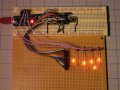This problem is considered resolved.Now to design a PIC Microcontroller version.

Last edited:

#### jayanthd

Joined Jul 4, 2015
904
Ok. I will make PIC version for you. Select the PIC you want. 40 pin 20 pin or 8 pin PIC. If lower pin count PIC then 2x or 4x HC595 shift regsisters have to be used which will make the circuit big and complex.

Also shiftregsiters can be used to display negative sign and decimal points.

Just decide whether you want to go for 40 pin PIC which will be cheaper and simpler or you want to go with shift registers based display.

My preference will be 40 pin or 44 pin or 64 pin SMD version of PIC18F46K22.

It will be very small circuit with just one single PIC.

Also tell me what max digits you want that is you want to display like

-123.4

or you want to display

-123.45

?